# The MDC Procedure

### Tests on Parameters

In general, the hypothesis to be tested can be written as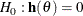where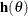is an r-by-1 vector-valued function of the parameters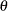given by the r expressions specified in the TEST statement.

Let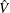be the estimate of the covariance matrix of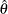. Letbe the unconstrained estimate ofand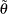be the constrained estimate ofsuch that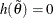. Let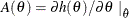Using this notation, the test statistics for the three kinds of tests are computed as follows:

• The Wald test statistic is defined as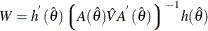The Wald test is not invariant to reparameterization of the model (Gregory and Veall 1985; Gallant 1987, p. 219). For more information about the theoretical properties of the Wald test, see Phillips and Park (1988).

• The Lagrange multiplier test statistic is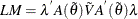where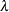is the vector of Lagrange multipliers from the computation of the restricted estimate.

• The likelihood ratio test statistic is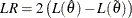whererepresents the constrained estimate ofand
is the concentrated log-likelihood value.

For each kind of test, under the null hypothesis the test statistic is asymptotically distributed as arandom variable with r degrees of freedom, where r is the number of expressions in the TEST statement. The p-values reported for the tests are computed from the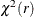distribution and are only asymptotically valid.

Monte Carlo simulations suggest that the asymptotic distribution of the Wald test is a poorer approximation to its small sample distribution than that of the other two tests. However, the Wald test has the lowest computational cost, since it does not require computation of the constrained estimate.

The following statements are an example of using the TEST statement to perform a likelihood ratio test:

   proc mdc;
model decision = x1 x2 / type=clogit
choice=(mode 1 2 3);
id pid;
test 0.5 * x1 + 2 * x2 = 0 / lr;
run;